### NCERT Solutions For Class 11 Math Chapter – 8 Miscelleneous Exercise

NCERT SolutoIns For Class 11 Math Chapter – 8 Miscelleneous Exercise

1. Find a, b and n in the expansion of (a + b)n if the first three terms of the expansion are 729, 7290 and 30375, respectively.

Solution:

We know that (r + 1)th term, (Tr+1), in the binomial expansion of (a + b)n is given by

Tr+1 = nCr an-t br

The first three terms of the expansion are given as 729, 7290 and 30375 respectively. Then we have,

T1 = nC0 an-0 b0 = an = 729….. 1

T2 = nC1 an-1 b= nan-1 b = 7290…. 2

T3 = nC2 an-2 b2 = {n (n -1)/2 }an-2 b2 = 30375……3

Dividing 2 by 1 we get

\frac{na^{n-1}b}{a^{n}} = \frac{7290}{729} \\ \frac{nb}{a} = 10

class="msupsub">nnan1b=7297290anb=10

Dividing 3 by 2 we get

\frac{n(n-1)a^{n-2}b^{2}}{2na^{n-1}b} = \frac{30375}{7290} \\ \frac{(n-1)b}{2a} = \frac{30375}{7290} \\ \frac{(n-1)b}{a} = \frac{30375}{7290} \times 2 =\frac{25}{3} \\ \frac{(nb)}{a} -\frac{b}{a} = \frac{25}{3} \\ 10 -\frac{b}{a} = \frac{25}{3} \\ \frac{b}{a} = 10 – \frac{25}{3} = \frac{5}{3}

From 4 and 5 we have

n. 5/3 = 10

n = 6

Substituting n = 6 in 1 we get

a6 = 729

a = 3

From 5 we have, b/3 = 5/3

b = 5

Thus a = 3, b = 5 and n = 76

2. Find a if the coefficients of x2 and x3 in the expansion of (3 + a x)9 are equal.

Solution: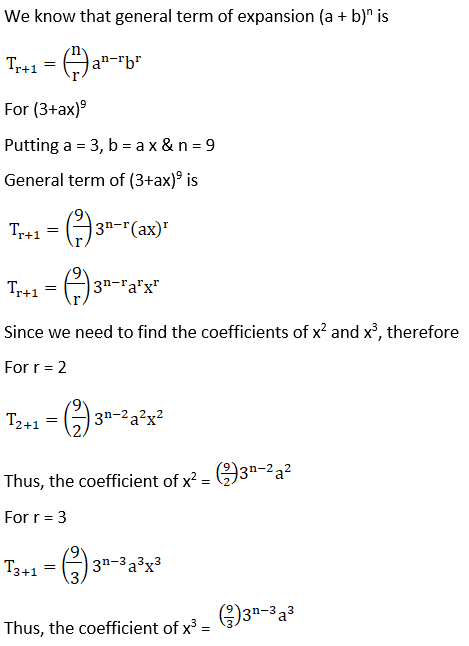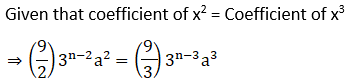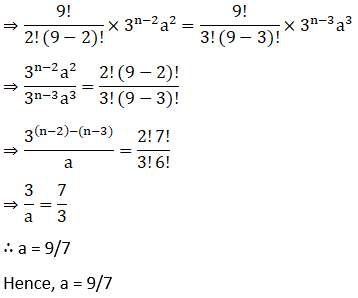3. Find the coefficient of x5 in the product (1 + 2x)6 (1 – x)7 using binomial theorem.

Solution:

(1 + 2x)6 = 6C6C1 (2x) + 6C2 (2x)2 + 6C3 (2x)3 + 6C4 (2x)4 + 6C5 (2x)5 + 6C6 (2x)6

= 1 + 6 (2x) + 15 (2x)2 + 20 (2x)3 + 15 (2x)4 + 6 (2x)5 + (2x)6

= 1 + 12 x + 60x2 + 160 x3 + 240 x4 + 192 x5 + 64x6

(1 – x)7 = 7C0 – 7C1 (x) + 7C(x)2 – 7C(x)3 + 7C(x)4 – 7C(x)5 + 7C(x)– 7C(x)7

= 1 – 7x + 21x2 – 35x3 + 35x4 – 21x5 + 7x6 – x7

(1 + 2x)6 (1 – x)7 = (1 + 12 x + 60x2 + 160 x3 + 240 x4 + 192 x5 + 64x6) (1 – 7x + 21x2 – 35x3 + 35x4 – 21x5 + 7x6 – x7)

192 – 21 = 171

Thus, the coefficient of x5 in the expression (1+2x)6(1-x)7 is 171.

4. If a and b are distinct integers, prove that a – b is a factor of an – bn, whenever n is a positive integer. [Hint write an = (a – b + b)n and expand]

Solution:

In order to prove that (a – b) is a factor of (an – bn), it has to be proved that

an – bn = k (a – b) where k is some natural number.

a can be written as a = a – b + b

an = (a – b + b)= [(a – b) + b]n

nC0 (a – b)n + nC1 (a – b)n-1 b + …… + n bn

an – bn = (a – b) [(a –b)n-1 + nC1 (a – b)n-1 b + …… + n bn]

an – bn = (a – b) k

Where k = [(a –b)n-1 + nC1 (a – b)n-1 b + …… + n bn] is a natural number

This shows that (a – b) is a factor of (an – bn), where n is positive integer.

5. Evaluate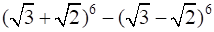Solution:

Using binomial theorem the expression (a + b)6 and (a – b)6, can be expanded

(a + b)6 = 6C0 a6 + 6C1 a5 b + 6C2 a4 b2 + 6C3 a3 b3 + 6C4 a2 b4 + 6C5 a b6C6 b6

(a – b)6C0 a6 – 6C1 a5 b + 6C2 a4 b2 – 6C3 a3 b3 + 6C4 a2 b4 – 6C5 a b6C6 b6

Now (a + b)6 – (a – b)6 =6C0 a6 + 6C1 a5 b + 6C2 a4 b2 + 6C3 a3 b3 + 6C4 a2 b4 + 6C5 a b6C6 b6 – [6C0 a6 – 6C1 a5 b + 6C2 a4 b2 – 6C3 a3 b3 + 6C4 a2 b4 – 6C5 a b6C6 b6]

Now by substituting a = √3 and b = √2 we get

(√3 + √2)6 – (√3 – √2)6 = 2 [6 (√3)5 (√2) + 20 (√3)3 (√2)3 + 6 (√3) (√2)5]

= 2 [54(√6) + 120 (√6) + 24 √6]

= 2 (√6) (198)

= 396 √6

6. Find the value of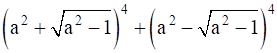Solution: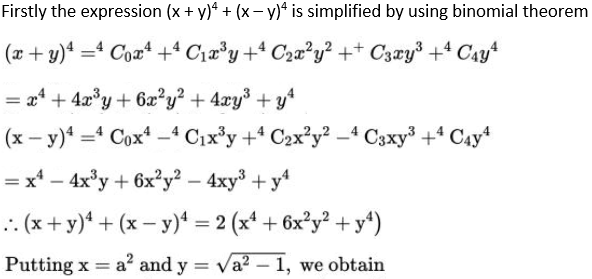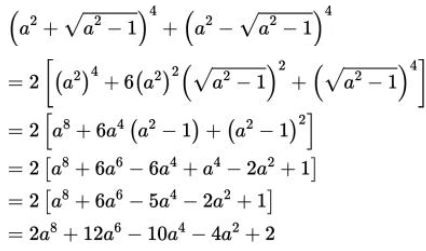7. Find an approximation of (0.99)5 using the first three terms of its expansion.

Solution:

0.99 can be written as

0.99 = 1 – 0.01

Now by applying binomial theorem we get

(o. 99)5 = (1 – 0.01)5

5C(1)5 – 5C(1)4 (0.01) + 5C(1)3 (0.01)2

= 1 – 5 (0.01) + 10 (0.01)2

= 1 – 0.05 + 0.001

= 0.951

8. Find n, if the ratio of the fifth term from the beginning to the fifth term from the end in the expansion of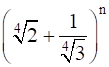is √6: 1

Solution: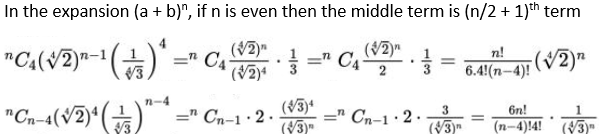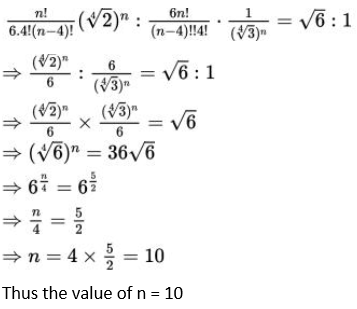9. Expand using Binomial Theorem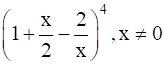Solution:

Using binomial theorem the given expression can be expanded as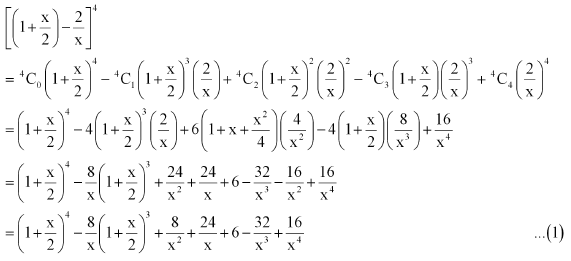Again by using binomial theorem to expand the above terms we get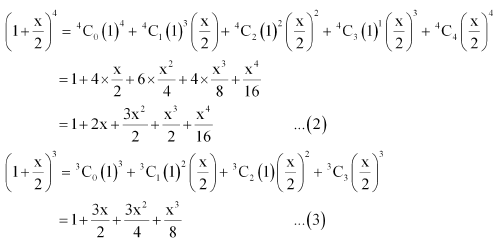From equation 1, 2 and 3 we get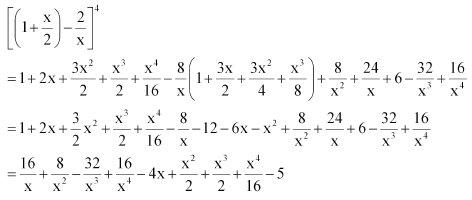10. Find the expansion of (3x2 – 2ax + 3a2)3 using binomial theorem.

Solution:

We know that (a + b)3 = a3 + 3a2b + 3ab2 + b3

Putting a = 3x2 & b = -a (2x-3a), we get

[3x2 + (-a (2x-3a))]3

= (3x2)3+3(3x2)2(-a (2x-3a)) + 3(3x2) (-a (2x-3a))2 + (-a (2x-3a))3

= 27x6 – 27ax(2x-3a) + 9a2x(2x-3a)2 – a3(2x-3a)3

= 27x6 – 54ax5 + 81a2x4 + 9a2x(4x2-12ax+9a2) – a[(2x)3 – (3a)3 – 3(2x)2(3a) + 3(2x)(3a)2]

= 27x6 – 54ax5 + 81a2x4 + 36a2x4 – 108a3x3 + 81a4x2 – 8a3x3 + 27a6 + 36a4x2 – 54a5x

= 27x6 – 54ax5+ 117a2x4 – 116a3x3 + 117a4x2 – 54a5x + 27a6

Thus, (3x2 – 2ax + 3a2)3

= 27x6 – 54ax5+ 117a2x4 – 116a3x3 + 117a4x2 – 54a5x + 27a6